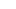Let’s take a look at how to analyze trends with moving average ribbons.

What is a moving average ribbon?

A moving average ribbon is a series of moving averages of different lengths plotted on a chart.

The basic idea behind “moving average ribbons” is that instead of using one or two moving averages on a chart, you are using a bunch of moving averages, usually between 6 to 16 moving averages (or more).

All are on the same chart.

Let’s take a look at an example…Traders can determine the strength of a trend by looking at the smoothness of the ribbon and identify key areas of support or resistance by looking at the price in relation to the ribbon.

## How to Set Up a Moving Average Ribbon

A common question is “How many moving averages do I use?”

It really depends on the trader.

Some traders like to use six to eight simple moving averages (SMA) set at 10-period intervals, such as the 10, 20, 30, 40, 50, and 60-day SMAs.

Other traders like to set up with SIXTEEN (or more) simple moving averages varying from a 50-day to a 200-day SMA and everything in between.

The argument for using longer-term MAs is that it gives a more accurate look at the overall trend.

Then other traders like to use exponential moving averages instead of simple moving averages.

So it’s really a matter of preference.

We can adjust the responsiveness of the moving average ribbon by:

• Changing the number of time periods used in the moving average
• Changing the type of moving average from a simple moving average (SMA) to an exponential moving average (EMA)

The shorter the number of periods used when selecting which MAs to add to your chart, the more sensitive the moving average ribbon is to slight price changes.

Using moving averages with larger numbers of periods (like 200) are less sensitive and smoother.